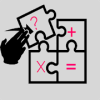LogiTap

LogiTapLogiTap - A fun offline math game. [详情]

LogiTap - A fun offline math game. The game is playable in 4 different levels - Very easy - where every correct answer gives 4 points, and every wrong answer takes 2 points. Easy - where every correct answer gives 10 points, and every wrong answer takes 5 points. Medium - where every correct answer gives 16 points, and every wrong answer takes 8 points. Hard - where every correct answer gives 22 points, and every wrong answer takes 11 points. The object of the game is getting the top score, while practicing adding and multiplying.

LogiTap游戏截图新游期待榜

1 一拳超人 角色 81240
2 拉结尔 角色 80191
3 辐射OL 策略 74108
4 方舟生存进化 冒险 71311
5 狂野飙车9 竞速 70529
6 梦幻西游3D 角色 68351
7 闪耀暖暖 角色 67732
8 长安幻世绘 卡牌 67309

猜你喜欢

@for=9@ @endfor@